## Calculating Asymmetric Grids Using Tuples

Cloud data source types: Oracle Essbase

Tuple calculation for asymmetric grids lets Oracle Smart View for Office users execute calculations for the specific scope of member selections in a grid or calculation script, avoiding over-calculation.

A calculation tuple is a way to represent a data slice of members, from one or more sparse dimensions, to be used in a calculation FIX statement.

By default, when multiple members from different dimensions are in a calculation FIX statement, or are part of the grid POV in Smart View, the calculation scope is all possible combinations (a Cartesian-product) of all member combinations from different dimensions. This can result in more calculations than are needed when working with asymmetric grids. With tuple calculations, the scope of calculations can be more precise and efficient.

Administrators set up tuple calculations in Essbase as they do other calculations that they create for users to run from Smart View, based on their specific data set and business needs.

As a Smart View user, you run tuple calculations for asymmetric grids as you would any other calculation. You may be asked to specify runtime prompts, depending on how your administrator set up the tuple calculation.

Note:

• You can run tuple calculations on asymmetric grids starting in Smart View release 11.1.2.5.800. Check that your Essbase data source supports calculation tuples.

• Administrators: Using the tuple calculation feature, you can define calculation scope as selected member tuples in an asymmetric grid. Special syntax in FIX statements helps you specify which tuples to calculate. See Calculate Selected Tuples in Using Oracle Essbase, for more information on tuple calculations.

In Smart View, to run a tuple calculation on an asymmetric grid, follow the instructions for running a calculation in Running Essbase Calculations.

Select a script written for tuple calculations on asymmetric grids. Your administrator can advise you which scripts you should run.

Example 8-3 Running Tuple Calculations on an Asymmetric Grid

The examples in this scenario are based on an example gallery template for tuple calculation. The gallery template is available in Essbase versions that support calculation tuples.

Start with the asymmetric grid shown below. The grid is asymmetric because we do not see the same member hierarchies in the row and column dimensions.

Figure 8-11 Starting Asymmetric Grid - All Data Values = 1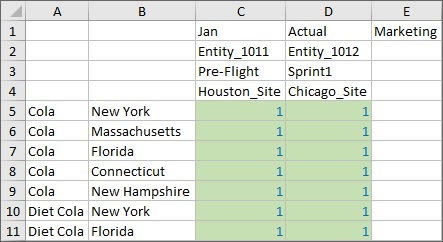The asymmetric grid above is a slice of this symmetric grid, where the hierarchy members are uniform across rows and columns:

Figure 8-12 Starting Symmetric Grid - All Data Values = 1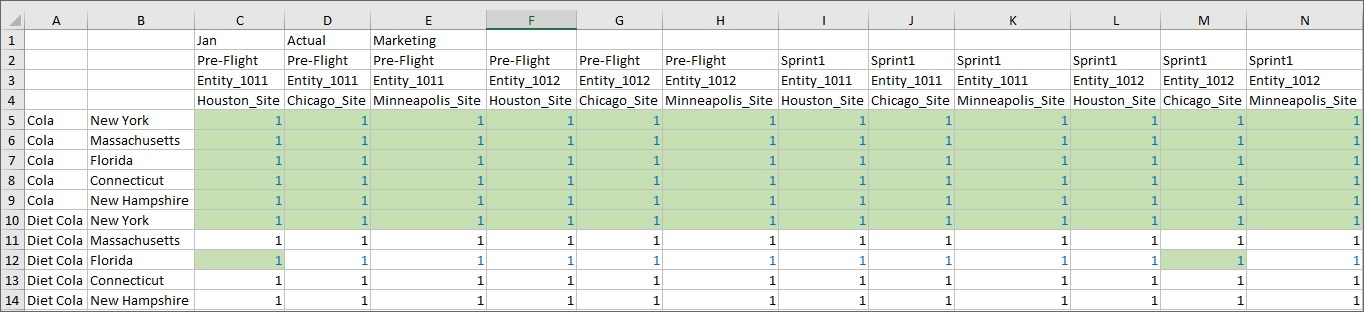We’ll show the result of running three different types of calculations on the asymmetric grid, and then the effect those calculations have on the symmetric grid.

The scripts are configured to increment the data cells by 1 each time they are run, with different results on the symmetric grid for each type of calculation.

The first script we’ll run calculates everything in the grid POV, not only the member combination shown in the grid below. In the asymmetric grid, after a refresh, all data cells now equal 2.

Figure 8-13 Asymmetric Grid After Running Default Calculation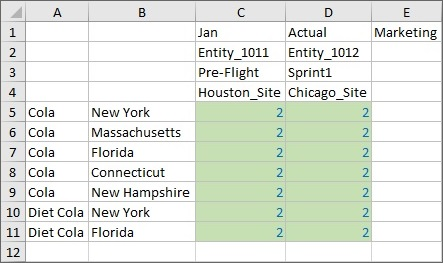We submit the cells in the asymmetric grid, then go to the symmetric grid and refresh it. Note that on refresh, every Entity, Version, Scenario and Site for the Product/Market combination is calculated, even though they aren't on the asymmetric grid. This is because, by default, absent a tuple calculation,Essbase calculates the Cartesian product of the combinations seen in the grid. The result is that all cells in the POV are incremented by 1, so you will see a value of 2 in all data cells in the symmetric grid:

Figure 8-14 Symmetric Grid After Running Default Calculation on Asymmetric Grid and Refresh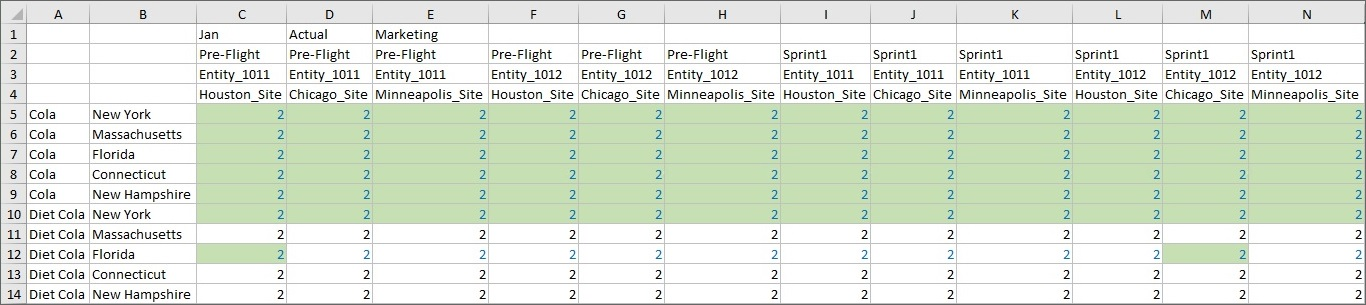Second, we’ll run a more limited calculation on the asymmetric grid, shrinking the calculation scope to Product and Market grid tuples so that fewer intersections are calculated. The Calculation Result message shows us the scope of this calculation:

Figure 8-15 Calculation Result Dialog After Running Tuple Calculation on All Product and Market Tuples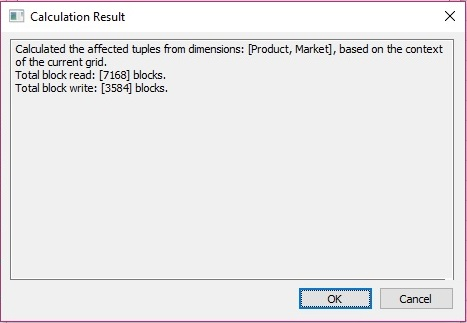Refresh, and all data cells in the asymmetric grid are incremented to 3. Submit the data on the grid.

Figure 8-16 Asymmetric Grid After Running Calculation on All Product and Market Tuples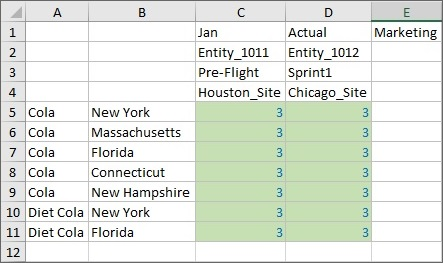In the symmetric grid, note on refresh that only the Product/Market tuples already included on the asymmetric grid are calculated, along with all Site/Version/Entity combinations. These are all the columns of rows 5 through 10, and row 12. These cells now show a data value of 3. The Product/Market tuples not shown in our asymmetric grid are not calculated in the symmetric grid; their data cell value is still 2. For this calculation, only Product and Market combinations were specified for tuples. The tuples and all members for unspecified dimensions (those not specified in the FIX statement) are calculated.

Figure 8-17 Symmetric Grid After Running Calculation on All Product and Market Tuples on Asymmetric Grid and Refresh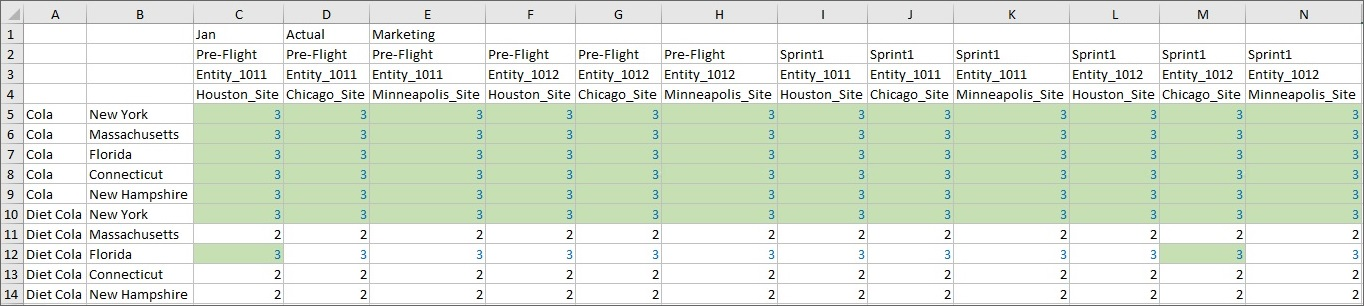Now run the last calculation, which is written to work only on the tuples shown in our asymmetric grid. The tuple calculation specifies that only the indicated Entity, Site, Market, Product, Version combinations on our asymmetric grid should be calculated. After running the calculation, the Calculation Result dialog shows an even smaller calculation scope:

Figure 8-18 Calculation Result Dialog After Running Calculation on Grid Product and Market Tuples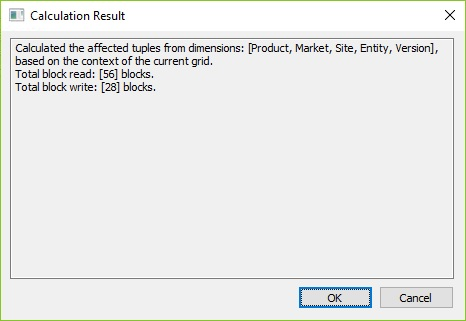Values in all cells in the asymmetric grid are incremented to 4. Submit the data on this grid.

Figure 8-19 Asymmetric Grid After Running Calculation on Grid Product and Market Tuples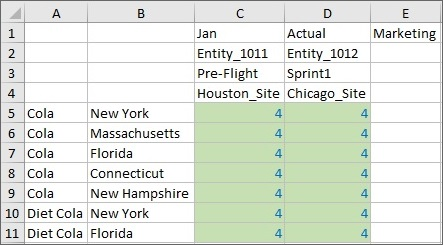Move to the symmetric grid and refresh. Remember, the tuple calculation specified that only the Entity, Site, Market, Product, Version combinations indicated on our asymmetric grid should be calculated. On the symmetric grid, you can see that only rows 5 through 10 and row 12 and columns C and M, are incremented to 4. These are the tuples that correspond to the data that was incremented in the asymmetric grid, and are highlighted in yellow below:

Figure 8-20 Symmetric Grid After Running Calculation on Grid Product and Market Tuples on Asymmetric Grid and Refresh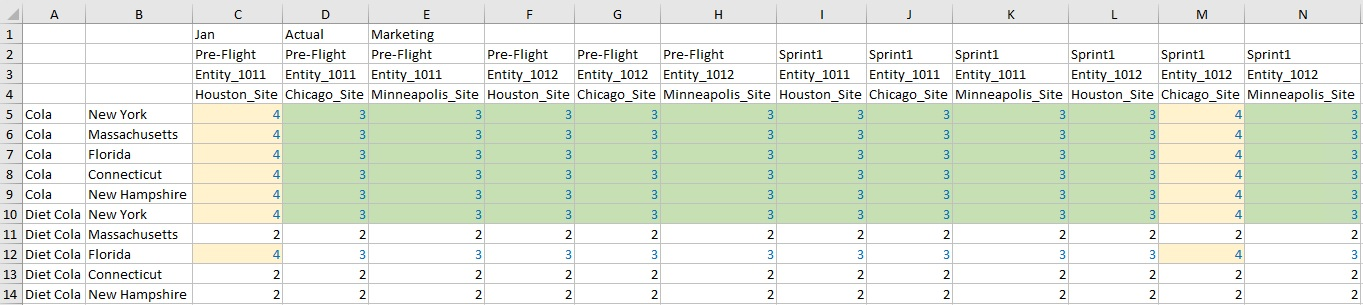The examples in this section showed you the effects of three calculations on an asymmetric grid and a symmetric grid. We looked at the result of each calculation on each type of grid. We learned that by selecting tuples, you can focus calculations in the active Smart View grid, limiting their scope to specific slices of data in your cube.